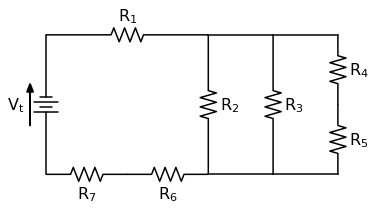## Solving a Circuit Diagram Problem with Python and SchemDraw

In this post we will solve a circuit diagram problem using a Python package called SchemDraw## Bar charts with error bars using Python, jupyter notebooks and matplotlib

Bar charts with error bars are useful in engineering to show the confidence or precision in a set of measurements or calculated values. Bar charts without error bars give the illusion that a measured or calculated value is known to high precision or high confidence. In this post, we will build a bar plot using Python, the statistics module and matplotlib. The plot will show the tensile strength of two different 3-D printer filament materials, ABS and HIPS. We will then add error bars to the plot based on the standard deviation of the data.

## Bar charts with error bars using Python and matplotlib

Bar charts with error bars are useful in engineering to show the confidence or precision in a set of measurements or calculated values. Bar charts without error bars give the illusion that a measured or calculated value is known to high precision or high confidence. In this post, we will build a bar plot using Python and matplotlib. The plot will show the coefficient of thermal expansion (CTE) of three different materials based on a small data set. Then we'll add error bars to this chart based on the standard deviation of the data.